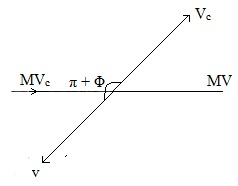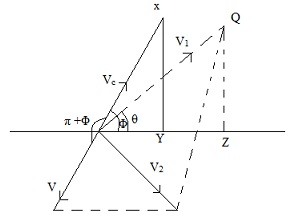#### Thermalisation, Physics tutorial

Introduction:

Here we will talk about details of nuclear reactions in which neutrons are projectiles. A good source of neutrons is  α-particles bombarding light elements.

4He2 + 9Be412C + 1 n 0 + Q

The α-particles are generally achieved from radium (Ra) in regular radioactive procedures. In such type of a reaction, up to 107 neutrons are ejected by means of energy 1-13MeV. Neutrons with this category of energy are referred as fast neutrons, for the reason of nuclear fission and large scale discharge of atomic energy; neutrons of energy in the neighborhood of 0.0025eV are desired. The neutrons are termed as thermal neutrons, Due to this is regarding the thermal energy 3 ⁄ 2 KT of molecules at thermal temperature. The method of slow down fast neutrons is known as "Thermalisation or Moderation".

Neutron Moderation:

The process of receiving fast neutrons to leisurely down to thermal neutron is considered as moderation or thermalisation. This is obtained by passing the fast neutrons by some suitable material termed as moderators. In like this way that neutrons are not loosed through absorption, but merely have their kinetic energy decreased gradually by elastic collision with the material's nuclei , such as good moderators are graphite and water. There are usually two frames of reference in the study of the dynamics of neutrons.

Laboratory frame:

In this frame, the target nucleus is at relaxed earlier than collision and it is reached by a projectile neutron with velocity Vo. After collision, nucleus is spread by angle θ and the target nucleus turns by angle θ′.The Centre Of Mass Frame:

This is theoretical but a extremely helpful technique in dealing with dynamics of moderation process. In this frame, the centre of mass of neutron and target nucleus is at rest and it is approached in reverse direction by the neutron and target nucleus. By principle of conservation of momentum,

mVc - MV = 0

or Vc = MV ⁄ m

Here Vc is velocity of neutron and V is velocity of target nucleus. After collision, neutron scatters off with angle Ø and nucleus pass all via (Ø + π ). To conserve momentum, velocity after collision remains unaffected.

XY = QZ

Sinθ = QZ / V1

V 1 sinθ = QZ

Vc Sin Ø  = XY

V1 ⁄Vc=sin Ø /sinθ

In laboratory framework, relative velocity = Vo as M is at rest. In centered mass frame, relative velocity = Vc + V while they move toward reverse direction.

These two relative velocities have to be equal in the 2 frames, in other words,

Vo = Vc + V

Combing equations and eliminating Vc

V = mV0/(M + m)

And Vc = MV0/(M + m) (eliminating V)

m/M ≈ 1/A

Here A is the mass number of nucleus. Therefore:

V = V0/1 + A

V0 = V(1 + A)

Such that Vc = V0A/1+A and Vc/V = V0A/1+A . 1+A/V0

V12 = V2 + Vc2 - 2VVCcos(1800 - Φ)

= V2 + Vc2 - 2VVC cosΦ

= V2 [1 + Vc2/V2 + 2VccosΦ/V]

V12 = V2(1 + A2 + 2AcosΦ)

So,

Let the incident energy of the neutron from the laboratory frame be given as:

E0 = 1/2mV02

After first collision, energy is reduced to:

E1 = = 1/2mV12

And fractional energy is given as:

E1/E2 = 1/2MV12/1/2MV02 = V12/V02

E1/E0 = (1 + A2 + 2AcosΦ)/(1 + A)2 (Fractional energy)

Cases of Interest:

1. Glancing of collision; (Φ = 0)

E1/E0 = (1 + A2 + 2AcosΦ)/(1 + A)2 = 1 + A2 +2A/1 + A2 + 2A = 1

This signifies E1 ≡ E0 thus neutron loses little or no energy on colliding with nucleus.

2.  Head on collision,( Φ =π)  Where, Cos π = - 1

E1/E0 = (1+A2-2A)/(1+A)2 = (A-1)2/(A+1)2

This kind of collision causes maximum energy loose through neutron such as for graphite that is a carbon allotrope and with its atomic mass, A = 12.   E1/E0 = 72 %

3.General case: 0 < Φ < π

Introducing α = [A-1/A+1]2

(ΔE)max = (E0 - E1)max

= E0(1 - E1/E0)

= E0(1 - α)

= (ΔE/E0)max = (1- α)

= 1-[A-1/A+1]2

= 4/A - 8/A2 + 12/A3 - 16/A4 + 20/A5

(ΔE/E0)max = 1-(1-1/A)2/(1+1/A)2

4/A[1-2/A + 3/A2 - 4/A3 + 5/A4]

A very significant point is that for material to act as good moderator, fractional energy loss should be large and from expression above, loss is smaller. From expression given above, it is seen that fractional energy loss (ΔE/E0)max is inversely proportional to atomic mass of moderator.

Passage of a Beam of a Neutron through a Moderating Material:

A flux of up to 108 fast neutrons to be moderated by several nuclei of moderator. The probability that single neutron will be scattered through angle Φ lying between Φ and dΦ such that energy of the neutron after scattering lies between E + dE. Range of energy is E1 = Eo for glancing angle collision and E1 = αE0 for head on collision. Probability that neutron will have energy E where αE0 is less than E and less than Eo(αE0<E<E0) after an arbitrary scattering is P(E). Energy between this range is:

E-αE0 = E0(1-α) so that P(E)dE = dE/E0(1-α)

Normalizing this probability

E1=α E0E0P(E)dE = αE0E0(dE/E0(1-α)) = 1

Average energy of neutron after series of scattering is probability that single collision will have energy E. Then, average energy is provided by:

<E> = [1/2[E0(1-α)][E2]αE0E0

<E> = 1/2[E0(1-α)

Where α = (A-1)2/(A+1)2

Average log energy decrement (ξ):

This term is introduced to get information about average number of collision which a fast neutron will make before its energy Eo is reduced to thermal energy Et, when Eo is reduced to E, log energy decrement is provided by:

logeE - logeE => loge(E0/E)

Average log decrement = <loge(E0/E)>

ζ = αE0E0 loge(E0/E)P(E)/dE

As αE0E0P(E)/dE = 1

Take x = E/E0

Then for E = αE0 where x = α

ζ = -1/1-α∫α1logxdx

= 1+ [α/1-α](logα)

By substituting α = (A-1/A+1)2

ζ = 1-(A-1)2/2A loge(A-1/A+1)

For A > 1, a convenient approximation

ζ = 2/A + 2/3

ζ α 1/A => n = 1/ζ loge(E0/E)

Where n is the number of collision needed to reduce fast neutron energy Eo to thermal energy Et.

Slowing down power Sp:

Effectiveness of moderating material is not only by log energy decrement but also by density of substance, i.e., number of colliding centers which a material has per unit volume.

Material should also have small absorption cross section, σa. Slowing down power is term that combines different parameters and it is expressed as:

Sp = ξNσa Or Sp = ξNaρσa/A

Where ρ is the density

A is atomic weight of moderator

σa = Absorption cross section

Neutron interaction:

All neutrons at the time of their birth are fast. In penetrating through matter, they suffer characteristics procedure of energy degradation or moderation. Probability of neutron interaction with the nucleus happening in moderating medium is cross-section signified by σ. This is estimated as effective area presented to neutron and it is defined in unit of barns.

Where 1 barn = 1 x 10-24cm2 (total part of material presented for interaction).

The total cross-section σt has several components:

σt = σet + σinelastic + σab + σf + ......

This is a sum of elastic, inelastic, absorption and fusion. All cross-sections are strongly energy dependent. The total cross-section μt is a microscopic quality. When multiplied by number N of absorber atoms per unit volume we have

Σ=σN

The removal of neutrons from a beam transversing a thickness t

I = Ioe-σNt Or I = I0eΣt

Io - initial intensity of beam neutron

Mean Free Path is distance travelled by neutron before collision

π = 1/Σ or 1/σN

Absorption:

As neutrons near thermal energy, the probability of capture by absorber nucleus increases i.e. absorption cross-section increases σab. For several absorbing nucleic, as neutron energy become very small (0.01 to 10,000eV), absorption cross-section is directly proportional to inverse of velocity and inversely proportional to energy.

σab α 1/v + 1/√E

Between range of energy (0.001 to 10,000eV) if the neutron enters reactor or start in reactor with certain energy Eo and having energy E after certain number of collision.

σ0/σ = √E/√E0

From absorption spectrum of neutrons inside reactor, resonance effect is observed.

Tutorsglobe: A way to secure high grade in your curriculum (Online Tutoring)